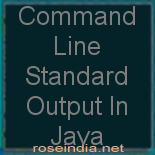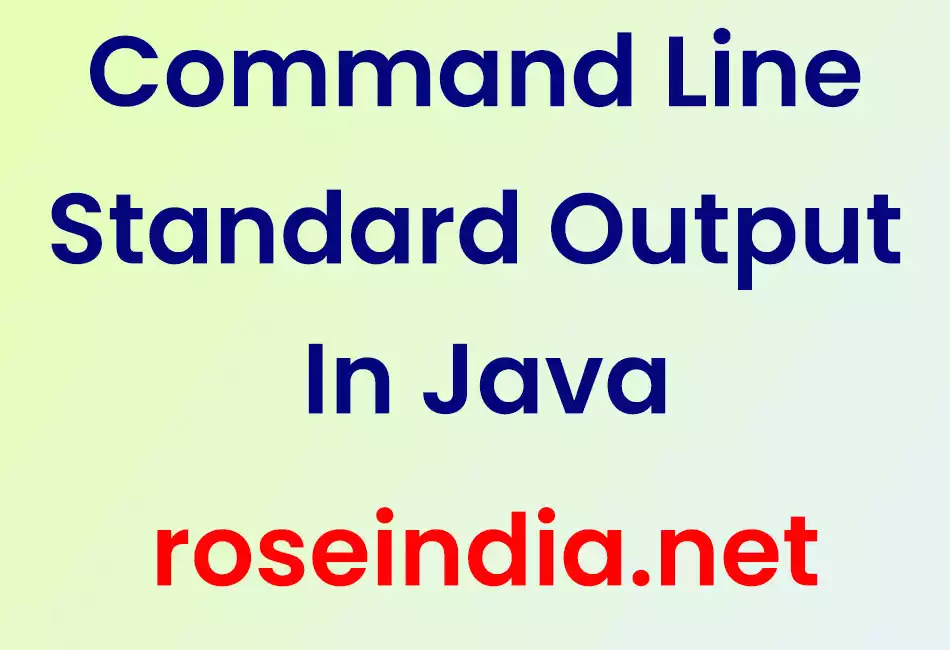# Command Line Standard Output In Java

In this section we will discuss about the Command Line Java IO Standard Output.### Command Line Standard Output In Java

In this section we will discuss about the Command Line Java IO Standard Output.## Command Line Standard Output In Java

In this section we will discuss about the Command Line Java IO Standard Output.

Standard streams, feature of various O/S, are written through standard output in Java. Standard streams can be written at the console, in a file, or at any output source. Java provides System.out to access the Standard Output. System.out is a byte stream.

System.out

In System.out, out is a public static final field defined as an object of PrintStream that specifies a standard output stream is open and ready for accepting output data. These output data can be displayed at the console, file or any other output destination depends upon the user or host environment. So, with the System.out we can use methods of PrintStream (discussed in the example given below).

public static final PrintStream out

Example

Here an example is being given which will demonstrate you about how standard output can be written at the console or any other dstination. In this example I have created a Java class named JavaSystemOutExample.java into which I have tried to write the output on console.

Source Code

JavaSystemOutExample.java

```public class JavaSystemOutExample
{
public static void main(String args[])
{
char c = 'd';
int i = 5;
String str = "Java Standard Output";
float f = 0.6f;
double d = 12.5;

System.out.println("char = "+c);
System.out.println("int = "+i);
System.out.println("string = "+str);
System.out.println("float = "+f);
System.out.println("double = "+d);

}
}
```

Output

When you will execute the above example you will get the output as follows :